Lakhmir Singh & Manjit Kaur Test: Motion

# Lakhmir Singh & Manjit Kaur Test: Motion - Class 9

Test Description

## 20 Questions MCQ Test Science Class 9 - Lakhmir Singh & Manjit Kaur Test: Motion

Lakhmir Singh & Manjit Kaur Test: Motion for Class 9 2023 is part of Science Class 9 preparation. The Lakhmir Singh & Manjit Kaur Test: Motion questions and answers have been prepared according to the Class 9 exam syllabus.The Lakhmir Singh & Manjit Kaur Test: Motion MCQs are made for Class 9 2023 Exam. Find important definitions, questions, notes, meanings, examples, exercises, MCQs and online tests for Lakhmir Singh & Manjit Kaur Test: Motion below.
Solutions of Lakhmir Singh & Manjit Kaur Test: Motion questions in English are available as part of our Science Class 9 for Class 9 & Lakhmir Singh & Manjit Kaur Test: Motion solutions in Hindi for Science Class 9 course. Download more important topics, notes, lectures and mock test series for Class 9 Exam by signing up for free. Attempt Lakhmir Singh & Manjit Kaur Test: Motion | 20 questions in 20 minutes | Mock test for Class 9 preparation | Free important questions MCQ to study Science Class 9 for Class 9 Exam | Download free PDF with solutions
 1 Crore+ students have signed up on EduRev. Have you?
Lakhmir Singh & Manjit Kaur Test: Motion - Question 1

### A vector quantity has

Lakhmir Singh & Manjit Kaur Test: Motion - Question 2

### The slope of the x - t graph is a measure of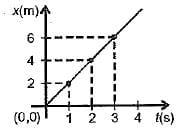Detailed Solution for Lakhmir Singh & Manjit Kaur Test: Motion - Question 2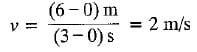Lakhmir Singh & Manjit Kaur Test: Motion - Question 3

### Odometer of automobiles records

Lakhmir Singh & Manjit Kaur Test: Motion - Question 4

Motion of bodies A and B is depicted by the x-t graph. Then which of the following is/are incorrect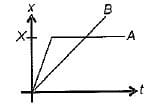A: A has uniform motion.
B: B has less velocity than A initially
C: B crosses A at X
D: A comes to rest at X

Detailed Solution for Lakhmir Singh & Manjit Kaur Test: Motion - Question 4

According to the graph given, the body A does not have uniform motion. An object is said to be in uniform motion when it covers equal distances in equal intervals of time. In this case, it accelerates to a particular velocity and remains the same continuously. Otherwise, it is evident that the velocity of B is lesser that A initially and the body B crosses A at X.
Hence, the statement A has uniform motion is not correct.

Lakhmir Singh & Manjit Kaur Test: Motion - Question 5

The speedometer of a car measures

Lakhmir Singh & Manjit Kaur Test: Motion - Question 6

​ From the x - t graph, one can draw the following conclusions ;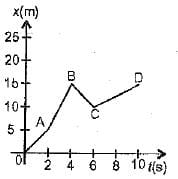A: vOA < vAB
B: vBC is negative
C: vCD is the least by magnitude
D: acceleration is uniform

Lakhmir Singh & Manjit Kaur Test: Motion - Question 7

If the displacement-time graph of a particle is parallel to the time axis, the velocity of the particle is

Lakhmir Singh & Manjit Kaur Test: Motion - Question 8

In a uniformly accelerated motion,
A: v - t graph is a straight line not parallel to t or v axis.
B: x - t graph is not a straight line.
C: Slope of v - t graph varies.
D: Slope of x - t graph is constant.

Lakhmir Singh & Manjit Kaur Test: Motion - Question 9

The velocity time graph of a moving particle is shown in Figure, The acceleration of the particle is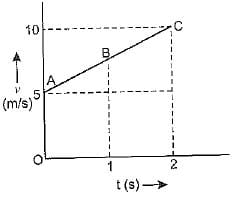Lakhmir Singh & Manjit Kaur Test: Motion - Question 10

If the velocity of a body is reducing, it is said to have

Lakhmir Singh & Manjit Kaur Test: Motion - Question 11

The velocity time (v - t) graph of a moving particle is shown in Figure. The acceleration is maximum for segment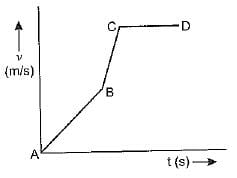Lakhmir Singh & Manjit Kaur Test: Motion - Question 12

One can conclude from the given x-t graph that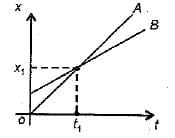Lakhmir Singh & Manjit Kaur Test: Motion - Question 13

The numerical ratio of displacement to distance for a moving object is

Lakhmir Singh & Manjit Kaur Test: Motion - Question 14

A body starting at a point, say A, reaches, say B, ahead in a straight line and returns back to A. Then there is

Lakhmir Singh & Manjit Kaur Test: Motion - Question 15

From the given v - t graph, it can be inferred that the object is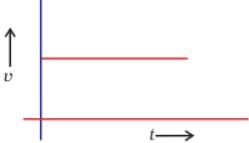Detailed Solution for Lakhmir Singh & Manjit Kaur Test: Motion - Question 15

In the given graph we find that the velocity is constant throughout, hence the object represented in the v - t graph is said to be in uniform motion.  Zero acceleration is also called uniform acceleration.

Lakhmir Singh & Manjit Kaur Test: Motion - Question 16

A body moves in a circle of radius 5 m with a speed of 5 ms-1. Then it has
A: an acceleration of 5 ms-2
B: an acceleration varying with direction alone

Lakhmir Singh & Manjit Kaur Test: Motion - Question 17

Four cars A, B, C and D are moving on a levelled road. Their distance versus time graphs are shown in figure.
Choose the correct statement.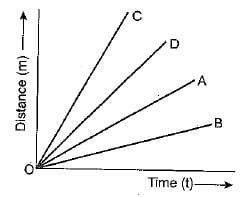Lakhmir Singh & Manjit Kaur Test: Motion - Question 18

The displacement of the body in 5 seconds from the beginning of the motion is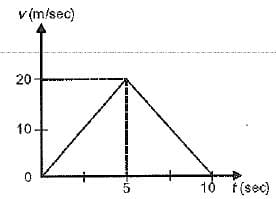Detailed Solution for Lakhmir Singh & Manjit Kaur Test: Motion - Question 18

Displacement = Area of ΔABC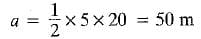Lakhmir Singh & Manjit Kaur Test: Motion - Question 19

Suppose a boy is enjoying a ride on a merry go round which is moving with a constant speed of 10 ms-1. It implies that the boy is

Detailed Solution for Lakhmir Singh & Manjit Kaur Test: Motion - Question 19

Acceleration is the measure of how fast the velocity changes. Velocity is a vector quantity and a change in velocity is due to  change in magnitude or change in direction or both. Here,  the boy is moving with constant speed but velocity changes and hence is supposed to be in accelerated motion.

Lakhmir Singh & Manjit Kaur Test: Motion - Question 20

The v-t graph shown here depicts the motion of A and B such that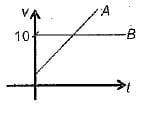## Science Class 9

66 videos|355 docs|97 tests
Information about Lakhmir Singh & Manjit Kaur Test: Motion Page
In this test you can find the Exam questions for Lakhmir Singh & Manjit Kaur Test: Motion solved & explained in the simplest way possible. Besides giving Questions and answers for Lakhmir Singh & Manjit Kaur Test: Motion, EduRev gives you an ample number of Online tests for practice

## Science Class 9

66 videos|355 docs|97 tests